# Three piles

100 kg of sugar we divide into three piles. The first pile is small. If we added to second 2 kg of sugar it would have 25% more sugar than the first pile. If we added to third pile 3 kg of sugar it would have 20% more sugar than the 2nd pile. How many kilograms of sugar are on each pile?

a =  28.64
b =  33.8
c =  37.56

### Step-by-step explanation:

a+b+c=100
b+2 = 1.25 a
c+3 = 1.20 b

a+b+c=100
b+2 = 1.25•a
c+3 = 1.20•b

a+b+c = 100
1.25a-b = 2
1.2b-c = 3

a = 716/25 = 28.64
b = 169/5 = 33.8
c = 939/25 = 37.56

Our linear equations calculator calculates it.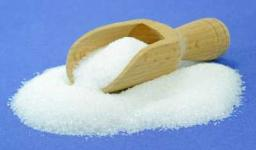Did you find an error or inaccuracy? Feel free to write us. Thank you!Tips to related online calculators
Do you have a linear equation or system of equations and looking for its solution? Or do you have a quadratic equation?

## Related math problems and questions:

• Acid evaporationHow many kilograms of water do we have to evaporate from 100 kg of 32% acid to make it 80% concentration?
• Fruit juice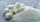Fruit juice contains 37.5% sugar. How many percents of sugar will be in the flavored mineral that we prepare from 100 grams of fruit juice and 1.4 liters of mineral? (1 liter = 1 kg)
• Barrel of oilBarrel of oil weighs 283 kg. When it mold 26% oil, weighed 216 kg. What is the mass of the empty barrel?
• GramsHow many grams of 5% solution do we need to add to 100g of 50% solution to get a 20% solution?
• Barrel 4Barrel of water weighs 63 kg. After off 75% water, the weight of the barrel with water is 21 kg. How many kg weigh empty barrel and how many kgs water in it?
• SugarSugar factory produced 127 tons of sugar in 1 day at 14% sugar content . How many tons of pure beet sugar factory processed in 1 day?
• Sugar 9150 kg of sugar beet yields 24 kg of sugar. From how many tons of sugar beet can be obtained three tons of sugar?
• Cargo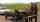The weight of the cargo car is 761 kg. The weight of the car is 23% of the total weight of car+cargo. What is the weight of the cargo load?
• Alcohol mixtureFrom 55% and 80% alcohol, we have to produce 0.2 kg of 60% alcohol. How many of them do we use in the solution?
• The water barrel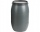The water barrel weighs 122 kg. If we pour 75% of the water out of it, it will weigh 35 kg. What is the weight of the barrel?
• SpiritFrom 55% and 80% spirit we would like to produce 0.2 kg of 60% spirit. How many of them we must use in a solution?
• Mixture of coffee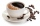They have to prepare a mixture of coffee in packing centres so that 1 kg costs 240 CZK. There are two types of coffee in stock at the price of 220 CZK per 1 kg and 300 CZK per 1 kg. How many kilograms of each species need to be mixed to prepare 50 kg of t
• MushroomsFresh mushrooms contain 88% water, dried 14% water. How many kilograms of fresh mushrooms should be collected to get 3 kg of dried?
• Collecting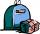For the first week of material collection events are picked up at the school 850 kg of paper and 305 kg of rags which represented 55% of total collection for 14 days. How many kilograms of secondary raw materials were handed over totally?
• SweetsWe want to prepare 5 kg of sweets for 150 CZK. We will mix cheaper candy: 1 kg for 120 CZK and more expensive candy: 1 kg per 240 CZK. How much of these two types of candy is necessary to prepare this mixture?
• Barrel 3Barrel with water weights 118 kg. When we get off 75% of water, it weights 35 kg. How many kg has an empty barrel?
• Dried fruit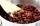The manufacturer produces a mixture of dried fruit. He purchased: 10kg pineapple for 200 Kc/kg 2kg papaya for 180 kc/kg 1kg of banana for 400 Kc/kg How many kgs of raisin for 80 Kc/kg must be put into the mix by the manufacturer so that the production pri